# SIunits

Library of type and unit definitions based on SI units according to ISO 31-1992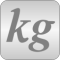# Package Contents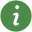UsersGuide User's Guide of SIunits Library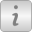Icons Icons for SIunits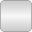Conversions Conversion functions to/from non SI units and type definitions of non SI units Angle (rad) SolidAngle (sr) Length (m) PathLength (m) Position (m) Distance (m) Breadth (m) Height (m) Thickness (m) Radius (m) Diameter (m) Area (m2) Volume (m3) Time (s) Duration (s) AngularVelocity (rad/s) AngularAcceleration (rad/s2) Velocity (m/s) Acceleration (m/s2) Period (s) Frequency (Hz) AngularFrequency (rad/s) Wavelength (m) Wavelenght (m) WaveNumber (m-1) CircularWaveNumber (rad/m) DampingCoefficient (s-1) LogarithmicDecrement (1/S) PhaseCoefficient (m-1) Damping (s-1) Mass (kg) Density (kg/m3) RelativeDensity SpecificVolume (m3/kg) LinearDensity (kg/m) SurfaceDensity (kg/m2) Momentum (kg.m/s) Impulse (N.s) AngularMomentum (kg.m2/s) AngularImpulse (N.m.s) MomentOfInertia (kg.m2) Inertia (kg.m2) Force (N) TranslationalDampingConstant (N.s/m) Weight (N) Torque (N.m) ElectricalTorqueConstant (N.m/A) MomentOfForce (N.m) RotationalSpringConstant (N.m/rad) RotationalDampingConstant (N.m.s/rad) Pressure (Pa) AbsolutePressure (Pa) BulkModulus (Pa) Stress (Pa) NormalStress (Pa) ShearStress (Pa) Strain LinearStrain ShearStrain VolumeStrain PoissonNumber ShearModulus (Pa) SectionModulus (m3) CoefficientOfFriction DynamicViscosity (Pa.s) KinematicViscosity (m2/s) SurfaceTension (N/m) Work (J) Energy (J) EnergyDensity (J/m3) Power (W) Efficiency MassFlowRate (kg/s) VolumeFlowRate (m3/s) MomentumFlux (N) AngularMomentumFlux (N.m) Absolute temperature (use type TemperatureDifference for relative temperatures) Temp_K (K) Temperature (K) Temp_C (degC) TemperatureSlope (K/s) PressureCoefficient (Pa/K) Compressibility (1/Pa) Heat (J) HeatFlowRate (W) HeatFlux (W/m2) DensityOfHeatFlowRate (W/m2) ThermalConductivity (W/(m.K)) CoefficientOfHeatTransfer (W/(m2.K)) SurfaceCoefficientOfHeatTransfer (W/(m2.K)) ThermalInsulance (m2.K/W) ThermalResistance (K/W) ThermalConductance (W/K) ThermalDiffusivity (m2/s) HeatCapacity (J/K) SpecificHeatCapacity (J/(kg.K)) SpecificHeatCapacityAtConstantPressure (J/(kg.K)) SpecificHeatCapacityAtConstantVolume (J/(kg.K)) SpecificHeatCapacityAtSaturation (J/(kg.K)) RatioOfSpecificHeatCapacities IsentropicExponent Entropy (J/K) EntropyFlowRate (J/(K.s)) SpecificEntropy (J/(kg.K)) Enthalpy (J) SpecificEnergy (J/kg) SpecificInternalEnergy (J/kg) SpecificEnthalpy (J/kg) SpecificGibbsFreeEnergy (J/kg) MassieuFunction (J/K) PlanckFunction (J/K) DerDensityByEnthalpy (kg.s2/m5) DerDensityByPressure (s2/m2) DerDensityByTemperature (kg/(m3.K)) DerEnthalpyByPressure (J.m.s2/kg2) DerEnergyByDensity (J.m3/kg) DerEnergyByPressure (J.m.s2/kg) DerPressureByDensity (Pa.m3/kg) DerPressureByTemperature (Pa/K) Current (A) CurrentSlope (A/s) Charge (C) VolumeDensityOfCharge (C/m3) SurfaceDensityOfCharge (C/m2) Voltage (V) VoltageSecond (V.s) Voltage second VoltageSlope (V/s) ElectricFluxDensity (C/m2) ElectricFlux (C) Capacitance (F) CapacitancePerArea (F/m2) Capacitance per area Permittivity (F/m) PermittivityOfVacuum (F/m) RelativePermittivity ElectricSusceptibility ElectricPolarization (C/m2) Electrization (V/m) ElectricDipoleMoment (C.m) CurrentDensity (A/m2) LinearCurrentDensity (A/m) MagneticFlux (Wb) MagneticVectorPotential (Wb/m) Inductance (H) CouplingCoefficient LeakageCoefficient Permeability (H/m) PermeabilityOfVacuum (H/m) RelativePermeability MagneticSusceptibility ElectromagneticMoment (A.m2) MagneticDipoleMoment (Wb.m) Magnetization (A/m) PoyntingVector (W/m2) Resistance (Ohm) Resistivity (Ohm.m) Conductivity (S/m) Reluctance (H-1) Permeance (H) PhaseDifference (rad) Impedance (Ohm) ModulusOfImpedance (Ohm) Reactance (Ohm) QualityFactor LossAngle (rad) Conductance (S) Admittance (S) Susceptance (S) ActivePower (W) ApparentPower (V.A) ReactivePower (var) PowerFactor First Order Temperature Coefficient Second Order Temperature Coefficient Transconductance (A/V2) InversePotential (1/V) RadiantEnergyDensity (J/m3) RadiantPower (W) RadiantEnergyFluenceRate (W/m2) RadiantIntensity (W/sr) Radiance (W/(sr.m2)) RadiantExtiance (W/m2) Irradiance (W/m2) Emissivity SpectralEmissivity DirectionalSpectralEmissivity LuminousFlux (lm) QuantityOfLight (lm.s) Luminance (cd/m2) LuminousExitance (lm/m2) Illuminance (lx) LightExposure (lx.s) LuminousEfficacy (lm/W) SpectralLuminousEfficacy (lm/W) LuminousEfficiency SpectralLuminousEfficiency CIESpectralTristimulusValues ChromaticityCoordinates SpectralAbsorptionFactor SpectralReflectionFactor SpectralTransmissionFactor SpectralRadianceFactor MolarAbsorptionCoefficient (m2/mol) RefractiveIndex StaticPressure (Pa) SoundPressure (Pa) VelocityOfSound (m/s) SoundEnergyDensity (J/m3) SoundPower (W) SoundIntensity (W/m2) AcousticImpedance (Pa.s/m3) SpecificAcousticImpedance (Pa.s/m) MechanicalImpedance (N.s/m) SoundPowerLevel (dB) DissipationCoefficient ReflectionCoefficient TransmissionCoefficient AcousticAbsorptionCoefficient LoudnessLevel (phon) Loudness (sone) LoundnessLevel (phon) Obsolete type, use LoudnessLevel instead! Loundness (sone) Obsolete type, use Loudness instead! RelativeAtomicMass RelativeMolecularMass NumberOfMolecules AmountOfSubstance (mol) MolarMass (kg/mol) MolarVolume (m3/mol) MolarDensity (mol/m3) MolarEnergy (J/mol) MolarInternalEnergy (J/mol) MolarHeatCapacity (J/(mol.K)) MolarEntropy (J/(mol.K)) MolarEnthalpy (J/mol) MolarFlowRate (mol/s) MassConcentration (kg/m3) MassFraction Concentration (mol/m3) VolumeFraction MoleFraction ChemicalPotential (J/mol) AbsoluteActivity PartialPressure (Pa) Fugacity (Pa) StandardAbsoluteActivity ActivityCoefficient ActivityOfSolute ActivityCoefficientOfSolute StandardAbsoluteActivityOfSolute ActivityOfSolvent OsmoticCoefficientOfSolvent StandardAbsoluteActivityOfSolvent OsmoticPressure (Pa) StoichiometricNumber Affinity (J/mol) MassOfMolecule (kg) MicrocanonicalPartitionFunction CanonicalPartitionFunction GrandCanonicalPartitionFunction MolecularPartitionFunction StatisticalWeight MeanFreePath (m) DiffusionCoefficient (m2/s) ThermalDiffusionRatio ThermalDiffusionFactor ChargeNumberOfIon FaradayConstant (C/mol) IonicStrength (mol/kg) DegreeOfDissociation MolarConductivity (S.m2/mol) TransportNumberOfIonic ProtonNumber NeutronNumber NucleonNumber MassOfElectron (kg) MassOfProton (kg) MassOfNeutron (kg) MagneticMomentOfParticle (A.m2) BohrMagneton (A.m2) NuclearMagneton (A.m2) GyromagneticCoefficient (A.m2/(J.s)) GFactorOfAtom GFactorOfNucleus MassExcess (kg) MassDefect (kg) RelativeMassExcess RelativeMassDefect PackingFraction BindingFraction MeanLife (s) LevelWidth (J) Activity (Bq) SpecificActivity (Bq/kg) DecayConstant (s-1) HalfLife (s) CrossSection (m2) AngularCrossSection (m2/sr) SpectralCrossSection (m2/J) SpectralAngularCrossSection (m2/(sr.J)) ParticleFluence (m-2) ParticleFluenceRate (s-1.m2) EnergyFluence (J/m2) EnergyFluenceRate (W/m2) CurrentDensityOfParticles (m-2.s-1) MassAttenuationCoefficient (m2/kg) MolarAttenuationCoefficient (m2/mol) TotalAtomicStoppingPower (J.m2) TotalMassStoppingPower (J.m2/kg) MeanMassRange (kg/m2) LinearIonization (m-1) TotalIonization Mobility (m2/(V.s)) IonNumberDensity (m-3) RecombinationCoefficient (m3/s) NeutronNumberDensity (m-3) NeutronSpeed (m/s) NeutronFluenceRate (s-1.m-2) TotalNeutronSourceDensity (s-1.m-3) SlowingDownDensity (s-1.m-3) ResonanceEscapeProbability Lethargy SlowingDownArea (m2) DiffusionArea (m2) MigrationArea (m2) NeutronYieldPerFission NeutronYieldPerAbsorption FastFissionFactor ThermalUtilizationFactor NonLeakageProbability Reactivity AbsorbedDose (Gy) DoseEquivalent (Sv) AbsorbedDoseRate (Gy/s) LinearEnergyTransfer (J/m) Kerma (Gy) KermaRate (Gy/s) Exposure (C/kg) ExposureRate (C/(kg.s)) ReynoldsNumber EulerNumber FroudeNumber GrashofNumber WeberNumber MachNumber KnudsenNumber StrouhalNumber FourierNumber PecletNumber RayleighNumber NusseltNumber BiotNumber StantonNumber FourierNumberOfMassTransfer PecletNumberOfMassTransfer GrashofNumberOfMassTransfer NusseltNumberOfMassTransfer StantonNumberOfMassTransfer PrandtlNumber SchmidtNumber LewisNumber MagneticReynoldsNumber AlfvenNumber HartmannNumber CowlingNumber BraggAngle (rad) OrderOfReflexion ShortRangeOrderParameter LongRangeOrderParameter DebyeWallerFactor CircularWavenumber (m-1) SpectralConcentration (s/m3) GrueneisenParameter MadelungConstant DensityOfStates (J-1/m-3) ResidualResistivity (Ohm.m) LorenzCoefficient (V2/K2) HallCoefficient (m3/C) SeebeckCoefficient (V/K) ThomsonCoefficient (V/K) RichardsonConstant (A/(m2.K2)) FermiEnergy (eV) GapEnergy (eV) ActivationEnergy (eV) HoleNumberDensity (m-3) DonorNumberDensity (m-3) EffectiveMass (kg) MobilityRatio ExchangeIntegral (eV) LandauGinzburgParameter FluxiodQuantum (Wb) TimeAging (1/s) ChargeAging (1/(A.s)) PerUnit DimensionlessRatio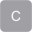ComplexCurrent Complex electrical currentComplexCurrentSlope Complex current slopeComplexCurrentDensity Complex electrical current densityComplexElectricPotential Complex electric potentialComplexPotentialDifference Complex electric potential differenceComplexVoltage Complex electrical voltageComplexVoltageSlope Complex voltage slopeComplexElectricFieldStrength Complex electric field strengthComplexElectricFluxDensity Complex electric flux densityComplexElectricFlux Complex electric fluxComplexMagneticFieldStrength Complex magnetic field strengthComplexMagneticPotential Complex magnetic potentialComplexMagneticPotentialDifference Complex magnetic potential differenceComplexMagnetomotiveForce Complex magnetomotive forceComplexMagneticFluxDensity Complex magnetic flux densityComplexMagneticFlux Complex magnetic fluxComplexReluctance Complex reluctanceComplexImpedance Complex electrical impedanceComplexAdmittance Complex electrical admittanceComplexPower Complex electrical powerComplexPerUnit Complex per unit

# Information

This information is part of the Modelica Standard Library maintained by the Modelica Association.

This package provides predefined types, such as Mass, Angle, Time, based on the international standard on units, e.g.,

```   type Angle = Real(final quantity = "Angle",